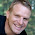## Monday, 20 November 2017

### JavaScript Regex list

JavaScript regex will help you to do client side validation very easily. In this article I will be sharing various regex examples. Below list contains regex with various conditions. I will be updating this list frequently with new regex condition. It will help everyone rather than spending time for creating new one, just open the link and select appropriate ones.

You can check the regex very easily using some of these site - regexpal.com, regex101.com. Or simply open the console and write like below,

``````
var regex  = 'yourregex pattern';
regex.exec('text to be tested')
```
```

If it returns null means validation conditions are not met, else it is success. You can use any of these to test below regex before using it. You can use this by simply putting along with html input. For example

``````
// html
<input type="text" required pattern="/^[A-Za-z]\w*\$/">

//angular
<input type="text" required ng-pattern="/^[A-Za-z]\w*\$/">

//or inside javascript file
var regex = "/^[A-Za-z]\w*\$/";
var _text = "validateMe";

if(regex.exec(_text) != null){
//success
}
```
```

#### JavaScript regex list.

Description Regex
• Only allows alphanumeric.
/^[a-z0-9]+\$/i
• Minimum 6 and maximum 20 characters.
• Must have at least one number
/^(?=.*\d).{6,20}\$/
• Alphanumeric.
• Allow some special characters (#, %, *, .)
/^[a-zA-Z0-9 #/()\\$%/{}/&*\[.]*\$/
• Only allows number.
• No decimal values
/^\d+\$/
• Alphanumeric.
• No special charactors but allow underscore
/^[A-Za-z]\w*\$/
• Minimum ten characters .
• At least one letter
• At least one number.
/^(?=.*[A-Za-z])(?=.*\d)[A-Za-z\d]{10,}\$/
• Minimum ten characters .
• At least one letter
• At least one number.
• At least one special character.
/^(?=.*[A-Za-z])(?=.*\d)(?=.*[\$@\$!%*#?&])[A-Za-z\d\$@\$!%*#?&]{10,}\$/
• Minimum ten characters .
• At least one uppercase letter
• At least one lowercase letter.
• At least one number
/^(?=.*[a-z])(?=.*[A-Z])(?=.*\d)[a-zA-Z\d]{10,}\$/
• Minimum ten characters.
• At least one uppercase letter
• At least one lowercase letter.
• At least one number
• At least one special character
/^(?=.*[a-z])(?=.*[A-Z])(?=.*\d)(?=.*[\$@\$!%*?&])[A-Za-z\d\$@\$!%*?&]{10,}/
• Minimum ten and maximum 20 characters.
• At least one uppercase letter
• At least one lowercase letter.
• At least one number
• At least one special character
/^(?=.*[a-z])(?=.*[A-Z])(?=.*\d)(?=.*[\$@\$!%*?&])[A-Za-z\d\$@\$!%*?&]{10,20}/
• Url validation (http://www.angulartutorial.net). ^(?:http(s)?:\/\/)?[\w.-]+(?:\.[\w\.-]+)+[\w\-\._~:/?#[\]@!\\$&'\(\)\*\+,;=.]+\$
• Minimum ten and maximum 20 characters.
• At least one uppercase letter
• At least one lowercase letter.
• At least one number
• At least one (any)special character or underscore
/^(?=.*[a-z])(?=.*[A-Z])(?=.*\d)(?=.*[_\W]).{12,20}\$/

In this article we have discussed about JavaScript regex and also added examples for regex with various conditions. I will be updating this list frequently.

#### 1 comment :

1.